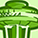Tip / Sign in to post questions, reply, level up, and achieve exciting badges. Know more

# MOSFET (Si/SiC) Forum DiscussionsLevel 1Level 1

# How to calculate junction temperature for a MOSFET?

Hi,

I use BSZ097N04 on a switching regulator design. I need to make sure the junction temperature is less than 150C.

the power go through the MOSFET have 66% duty cycle. I can only find Zth(jc) plot in the data sheet and curves are only  available with less than 50% duty cycle. However i need to know Zth(ja), as it specified PCB cooling size, to calculate junction temperature.

1. can you tell me how to calculate junction temperature in my case?

2. for Zth(jc) (junction-to-case) this case is top side or bottom side of package?

Thanks,

Tony

1 SolutionModeratorModerator

# Re: How to calculate junction temperature for a MOSFET?

Hello Tony,
Thank you for posting on Infineon Community.

1. The best way to calculate junction temperature is  by means of simulation using the equivalent thermal model provided in the datasheet and using the following application notes  :
Infineon-Application_note_Dynamic_thermal_behavior_of_MOSFETs
PowerMOSFET_SimulationModels

Alternatively,if you intend to experiment with the device temperature, the quickest approach is to use a thermocouple
or a thermal camera to estimate the hottest device temperature, which is usually in correspondence with where the chip is located within the package.

2. Zth(jc) = (Tj - Tc)/P, where Tj is junction temperature,Tc is case temperature and P is power dissipated from junction to case. Tj is the junction temperature (on a standard test chip, it is located at the top center of the chip), Tc is the case temperature, normally measured at the top, center of the test package.

Regards,
AnshikaModeratorModerator

# Re: How to calculate junction temperature for a MOSFET?

Hello Tony,
Thank you for posting on Infineon Community.

1. The best way to calculate junction temperature is  by means of simulation using the equivalent thermal model provided in the datasheet and using the following application notes  :
Infineon-Application_note_Dynamic_thermal_behavior_of_MOSFETs
PowerMOSFET_SimulationModels

Alternatively,if you intend to experiment with the device temperature, the quickest approach is to use a thermocouple
or a thermal camera to estimate the hottest device temperature, which is usually in correspondence with where the chip is located within the package.

2. Zth(jc) = (Tj - Tc)/P, where Tj is junction temperature,Tc is case temperature and P is power dissipated from junction to case. Tj is the junction temperature (on a standard test chip, it is located at the top center of the chip), Tc is the case temperature, normally measured at the top, center of the test package.

Regards,
Anshika